Do you like the article?
Share it with others -
post a link to it!
Use new possibilities of MetaTrader 5

# Sending Trading Signals in a Universal Expert Advisor

17 July 2007, 13:41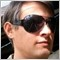0
4 178

### Introduction

Some time ago I decided to try to universalize and unitize a process of developing Expert Advisors in the topic "The Development of a Universal Expert Advisor". This implied working out a certain standard of developing main unit-bricks, which later could be used to construct Expert Advisors like from parts of an construction kit. Partly this task was implemented. I offered a structure of a universal EA and an idea of a universal use of signals of different indicators. In this article I will continue this work - I will try to universalize the process of forming and sending trading signals and item management in Expert Advisors. In this context an item means an operation - BUY or SELL, all later discussion will refer to such operations. This article does not describe pending orders BUYLIMIT, BUYSTOP, SELLLIMIT and SELLSTOP, but at the end of the article I will show, that this my approach can be easily applied on them.

### Classification of Trading Signals

There are several types of trading signals:

1. Buy;
2. Sell;
3. Additional buy (averaging);
4. Additional sell (averaging);
5. Full buy close;
6. Full sell close;
7. Partial buy close;
8. Partial sell close.

If we transpose the decision about averaging and partial closing from the unit of trading signals formation into the positions and orders management unit, this list will be reduced to the following:

1. Buy;
2. Sell;
3. Close buy;
4. Close sell;
5. Do nothing (normal operation of EA requires such signal).

Assuming this, the operation scheme of EA should be the following:

1. A signal item creates a trading signal;
2. A trading signal enters the positions management unit, which decides on new openings, averaging, partial or full closing and sends the shortened trading signal into the program unit of trading signals processing;
3. The unit of trading signals processing directly performs trading operations.

In this article I would like to dwell on different ways of forming trading signals and ways of their sending to positions management unit, i.e. the interface between the units, mentioned in points 1 and 2.

### Swing Trading on One Position

The meaning of such trading is the following: before opening a new position, the previous one should be closed. The new position is opposite to the previous one. If it was buy, we open sell and vice verse. In this case moments of position opening and closing concur in time, that is why closing signals can be omitted. So the implementation of swing trading requires sending only three trading signals: buy, sell and do nothing. These signals can be sent using one int variable. For example:

1 - buy;
0 - do nothing;
-1 - sell.

Then the part of analysing market situation and creating a trading signal can be written in a separate function, for example GetTradeSignal(),

```//+----------------------------------------------------------------------------+
//|  Returns trading signal:                                                   |
//|     1 - buy                                                                |
//|     0 - do nothing                                                         |
//|    -1 - sell                                                               |
//|  Parameters:                                                               |
//|    sym - name of the instrument ("" - current symbol)                      |
//|    tf  - timeframe                ( 0 - current timeframe)                 |
//+----------------------------------------------------------------------------+
int GetTradeSignal(string sym="", int tf=0)
{
int bs=0;
if (sym=="") sym=Symbol();

// Block of analysis assigning a value to the variable bs

return(bs);
}
//+----------------------------------------------------------------------------+```

which returns the above mentioned integer values. Activation of this function is easiest done directly in the positions management unit.

```//+----------------------------------------------------------------------------+
//|  Positions management                                                      |
//+----------------------------------------------------------------------------+
void ManagePositions()
{
double sl=0, tp=0;
int    bs=GetTradeSignal();

if(bs>0)
{
if(ExistPositions("", OP_SELL))
ClosePositions("", OP_SELL);
if(!ExistPositions("", OP_BUY))
{
if(StopLoss!=0)
sl=Ask-StopLoss*Point;
if(TakeProfit!=0)
tp=Ask+TakeProfit*Point;
OpenPosition("", OP_BUY, sl, tp);
}
}
if(bs<0)
{
if(ExistPositions("", OP_BUY))
ClosePositions("", OP_BUY);
if(!ExistPositions("", OP_SELL))
{
if(StopLoss!=0)
sl=Bid+StopLoss*Point;
if(TakeProfit!=0)
tp=Bid-TakeProfit*Point;
OpenPosition("", OP_SELL, sl, tp);
}
}
}
//+----------------------------------------------------------------------------+```

In this case a single local variable bs of the integer type acts as a linker between two program units. The full text of the example source code for swing trading by one position is located in the file e-SampleSwing.mq4.

### Simple Trading on One Position

This case is a little more difficult. Though in the market there is only one position at each point of time, its closing is not connected with the opening of another one. That is why a successful positions management requires using all five signals: buy, sell, close buy position, close sell position, and do nothing. They can be sent in one integer type variable with the following values assigned:

• 2 - close sell position;
• 1 - buy;
• 0 - do nothing;
• -1 - sell;
• -2 - close buy position.

Positions management unit can be written in one function:

```//+----------------------------------------------------------------------------+
//|  Positions management                                                      |
//+----------------------------------------------------------------------------+
void ManagePositions()
{
double sl=0, tp=0;
int    bs=GetTradeSignal();

if(ExistPositions())
{
if(bs==2)
ClosePositions("", OP_SELL);
if(bs==-2)
ClosePositions("", OP_BUY);
}
else
{
if(bs==1)
{
if(StopLoss!=0)
sl=Ask-StopLoss*Point;
if(TakeProfit!=0)
tp=Ask+TakeProfit*Point;
OpenPosition("", OP_BUY, sl, tp);
}
if(bs==-1)
{
if(StopLoss!=0)
sl=Bid+StopLoss*Point;
if(TakeProfit!=0)
tp=Bid-TakeProfit*Point;
OpenPosition("", OP_SELL, sl, tp);
}
}
}
//+----------------------------------------------------------------------------+```

The full text of the example source code for simple trading by one position is located in the file e-SampleSimple.mq4.

The main disadvantage of sending a trading signal in one variable is that you cannot send several signals simultaneously (disadvantages of a serial interface) - for example, you cannot open in both directions, or open with a simultaneous closing of an existing position, or close all positions. Partially this disadvantage can be eliminated by dividing a signal into two integers.

There are three ways of dividing a signal:

1. Open in one variable, close in another one. You can combine opening and closing, i.e. organize swing trading, but you cannot open in both directions or close counter-direction positions;
2. BUY positions (open and close) are in one variable, SELL positions (open and close) are in another one. You can open positions in both directions and close all positions at once, but you cannot simultaneously open and close, for example, buy or sell position, i.e. you cannot reopen;
3. Open BUY and close SELL in one variable, open SELL and close BUY in another. You can open in both directions and close all positions, but you cannot organise swing trading.

Let us try to implement the second variant, because reopening is rather rare. No one wants to lose money at spread. The second variant can be implemented in several ways.

1. Two functions return values into two local variables. One function creates signals for buying, the second one - for selling.

```//+----------------------------------------------------------------------------+
//|  Returns trading signal for long positions:                                |
//|     1 - buy                                                                |
//|     0 - do nothing                                                         |
//|    -1 - close BUY                                                          |
//|  Parameters:                                                               |
//|    sym - name of the instrument ("" - current symbol)                      |
//|    tf  - timeframe                ( 0 - current timeframe)                 |
//+----------------------------------------------------------------------------+
int GetTradeSignalBuy(string sym="", int tf=0)
{
int bs=0;
if(sym=="")
sym=Symbol();

// Block of analysis assigning a value to the variable bs

return(bs);
}

//+----------------------------------------------------------------------------+
//|  Returns trading signal for short positions:                               |
//|     1 - sell                                                               |
//|     0 - do nothing                                                         |
//|    -1 - close SELL                                                         |
//|  Parameters:                                                               |
//|    sym - name of the instrument ("" - current symbol)                      |
//|    tf  - timeframe                ( 0 - current timeframe)                 |
//+----------------------------------------------------------------------------+
int GetTradeSignalSell(string sym="", int tf=0)
{
int bs=0;
if(sym=="")
sym=Symbol();

// Block of analysis assigning a value to the variable bs

return(bs);
}

//+----------------------------------------------------------------------------+
//|  Positions management                                                      |
//+----------------------------------------------------------------------------+
void ManagePositions()
{
double sl=0, tp=0;
int    bs=GetTradeSignalBuy();
int    ss=GetTradeSignalSell();

if(ExistPositions())
{
if(bs<0)
ClosePositions("", OP_BUY);
if(ss<0)
ClosePositions("", OP_SELL);
}
if(!ExistPositions())
{
if(bs>0)
{
if(StopLoss!=0)
sl=Ask-StopLoss*Point;
if(TakeProfit!=0)
tp=Ask+TakeProfit*Point;
OpenPosition("", OP_BUY, sl, tp);
}
if(ss>0)
{
if(StopLoss!=0)
sl=Bid+StopLoss*Point;
if(TakeProfit!=0)
tp=Bid-TakeProfit*Point;
OpenPosition("", OP_SELL, sl, tp);
}
}
}
//+----------------------------------------------------------------------------+```

This is a simple and convenient way to create and send a trading signal, but a signal unit is divided into two functions which would probably slow down its operation.

2. Two global variables acquire values in one function.

```//+----------------------------------------------------------------------------+
BuySignal=0;
SellSignal=0;

//+----------------------------------------------------------------------------+
//|  Creates trading signals.                                                  |
//|  Parameters:                                                               |
//|    sym - name of the instrument ("" - current symbol)                      |
//|    tf  - timeframe              ( 0 - current timeframe)                   |
//+----------------------------------------------------------------------------+
void FormTradeSignals(string sym="", int tf=0)
{
if(sym=="") sym=Symbol();

// Block of analysis assigning a value to the variable BuySignal and SellSignal
}

//+----------------------------------------------------------------------------+
//|  Positions management                                                      |
//+----------------------------------------------------------------------------+
void ManagePositions()
{
double sl=0, tp=0;

FormTradeSignals();
if(ExistPositions())
{
if(BuySignal<0)
ClosePositions("", OP_BUY);
if(SellSignal<0)
ClosePositions("", OP_SELL);
}
if(!ExistPositions())
{
if(BuySignal>0)
{
if(StopLoss!=0)
sl=Ask-StopLoss*Point;
if(TakeProfit!=0)
tp=Ask+TakeProfit*Point;
OpenPosition("", OP_BUY, sl, tp);
}
if(SellSignal>0)
{
if(StopLoss!=0)
sl=Bid+StopLoss*Point;
if(TakeProfit!=0)
tp=Bid-TakeProfit*Point;
OpenPosition("", OP_SELL, sl, tp);
}
}
}
//+----------------------------------------------------------------------------+```

It is clear from this source code, that realization with global variables does not differ much from the first method. The apparent advantage is that the signal mode is in one function.

3. Two local variables are passed by reference to one function.

```//+----------------------------------------------------------------------------+
//|  Returns trading signals.                                                  |
//|  Parameters:                                                               |
//|    bs  - BUY signal                                                        |
//|    ss  - SELL signal                                                       |
//|    sym - name of the instrument ("" - current symbol)                      |
//|    tf  - timeframe                ( 0 - current timeframe)                 |
//+----------------------------------------------------------------------------+
void GetTradeSignals(int& bs, int& ss, string sym="", int tf=0)
{
if(sym=="")
sym=Symbol();

// Block of analysis assigning a value to variables bs and ss
}

//+----------------------------------------------------------------------------+
//|  Positions management                                                      |
//+----------------------------------------------------------------------------+
void ManagePositions()
{
double sl=0, tp=0;
int    bs=0, ss=0;

GetTradeSignals(bs, ss);
if(ExistPositions())
{
if(bs<0)
ClosePositions("", OP_BUY);
if(ss<0)
ClosePositions("", OP_SELL);
}
if(!ExistPositions())
{
if(bs>0)
{
if(StopLoss!=0)
sl=Ask-StopLoss*Point;
if(TakeProfit!=0)
tp=Ask+TakeProfit*Point;
OpenPosition("", OP_BUY, sl, tp);
}
if(ss>0)
{
if(StopLoss!=0)
sl=Bid+StopLoss*Point;
if(TakeProfit!=0)
tp=Bid-TakeProfit*Point;
OpenPosition("", OP_SELL, sl, tp);
}
}
}
//+----------------------------------------------------------------------------+```

4. An array of two elements. If it is global, it is initialized inside the function. If it is local, it is passed by reference. Everything is easy here - one array instead of two variables. Global elements and local elements by reference were discussed earlier. Nothing new.

And, finally, we can organize a full-valued parallel interface sending trading signals, dividing it into four variables. For positions management each signal has two states: exists or not. That is why we should better deal with variables of logical type. You can combine signals, sending them using four variables, limitless. Here is an example of a code with an array of four elements of logical type.

```//+----------------------------------------------------------------------------+
//|  Returns trading signals.                                                  |
//|  Parameters:                                                               |
//|    ms  - array of signals                                                  |
//|    sym - name of the instrument ("" - current symbol)                      |
//|    tf  - timeframe                ( 0 - current timeframe)                 |
//+----------------------------------------------------------------------------+
void GetTradeSignals(bool& ms[], string sym="", int tf=0)
{
if(sym=="")
sym=Symbol();

// Block of analysis filling ms array:
// ms=True;   // Buy
// ms=True;   // Sell
// ms=True;   // Close Buy
// ms=True;   // Close Sell
}

//+----------------------------------------------------------------------------+
//|  Positions management                                                      |
//+----------------------------------------------------------------------------+
void ManagePositions()
{
bool   ms={False, False, False, False};
double sl=0, tp=0;

GetTradeSignals(ms);
if(ExistPositions())
{
if(ms)
ClosePositions("", OP_BUY);
if(ms)
ClosePositions("", OP_SELL);
}
if(!ExistPositions())
{
if(ms)
{
if(StopLoss!=0)
sl=Ask-StopLoss*Point;
if(TakeProfit!=0)
tp=Ask+TakeProfit*Point;
OpenPosition("", OP_BUY, sl, tp);
}
if(ms)
{
if(StopLoss!=0)
sl=Bid+StopLoss*Point;
if(TakeProfit!=0)
tp=Bid-TakeProfit*Point;
OpenPosition("", OP_SELL, sl, tp);
}
}
}
//+----------------------------------------------------------------------------+```

Implementation with local variables, passed by reference to one function, and with global variables, initialized inside one function, should not cause any problems. However, initialization of four local variables by values, returned by four different functions, is hardly reasonable.

### Supporting the Main Position

When a signal comes, one position is opened. It becomes the main position. All other signal entries against the main position are ignored. Additional positions are opened on signals, corresponding with the main position. At the closing of the main position, all additional positions are also closed.

Sending trading signals for this tactics can be implemented using one, two or four variables. All this was described earlier. The difficulty, you can meet, is providing the connection: one signal - one position. In case of trading on one position this question was solved by a simple check of the position existence using the function ExistPositions(). If there is a position, entrance signal is omitted, no position - entrance signal is performed.

So, there are different ways out:

1. Providing a pause between entrances. The easiest realization;
2. One bar - one entrance. A variety of the first way. The realization is also easy;
3. Numerating signals and controlling arrays of signals and tickets of orders. This is the most difficult implementation with a doubtful reliability.

This is the example of a code for the first method:

```//+----------------------------------------------------------------------------+
extern int PauseBetweenEntrys=3600;    // Pause between entrances in seconds

//+----------------------------------------------------------------------------+
//|  Positions management                                                      |
//+----------------------------------------------------------------------------+
void ManagePositions()
{
bool   ms={False, False, False, False};
double sl=0, tp=0;

GetTradeSignals(ms);
if(ExistPositions())
{
if(ms)
ClosePositions("", OP_BUY);
if(ms)
ClosePositions("", OP_SELL);
}
if(SecondsAfterOpenLastPos()>=PauseBetweenEntrys)
{
if(ms)
{
if(StopLoss!=0)
sl=Ask-StopLoss*Point;
if(TakeProfit!=0)
tp=Ask+TakeProfit*Point;
OpenPosition("", OP_BUY, sl, tp);
}
if(ms)
{
if(StopLoss!=0)
sl=Bid+StopLoss*Point;
if(TakeProfit!=0)
tp=Bid-TakeProfit*Point;
OpenPosition("", OP_SELL, sl, tp);
}
}
}

//+----------------------------------------------------------------------------+
//|  Returns number of seconds after the last position is opened.              |
//|  Parameters:                                                               |
//|    sym - name of the instrument ("" - current symbol)                      |
//|    op  - operation              (-1 - any position)                        |
//|    mn  - MagicNumber            (-1 - any magic number)                    |
//+----------------------------------------------------------------------------+
datetime SecondsAfterOpenLastPos(string sym="", int op=-1, int mn=-1)
{
datetime oot;
int      i, k=OrdersTotal();

if(sym=="") sym=Symbol();
for(i=0; ik; i++)
{
if(OrderSelect(i, SELECT_BY_POS, MODE_TRADES))
{
if(OrderSymbol()==sym)
{
if(OrderType()==OP_BUY || OrderType()==OP_SELL)
{
if(op0 || OrderType()==op)
{
if(mn0 || OrderMagicNumber()==mn)
{
if(ootOrderOpenTime())
oot=OrderOpenTime();
}
}
}
}
}
}
return(CurTime()-oot);
}
//+----------------------------------------------------------------------------+```

The full text of the example source code for the tactics with supporting the main position is located in the file e-SampleMain.mq4.

### Swing Trading with Averaging

When an entrance signal comes, one position is opened. Based on all further signals in the direction of the first position, new additional positions are opened. When a signal comes against the existing positions, all positions are closed and one position is opened in the direction of the signal. The trading signal is repeated.

Sending and execution of trading signals for swing trading on one position has been discussed. Let us adapt this example to implement the option of opening additional positions. To provide the connection one signal - one position, let us use the restriction of entrance possibility by a time period of one bar. One bar - one entrance.

```//+----------------------------------------------------------------------------+
//|  Positions management                                                      |
//+----------------------------------------------------------------------------+
void ManagePositions()
{
double sl=0, tp=0;
int    bs=GetTradeSignal();

if(bs>0)
{
if(ExistPositions("", OP_SELL))
ClosePositions("", OP_SELL);
if(NumberOfBarLastPos()>0)
{
if(StopLoss!=0)
sl=Ask-StopLoss*Point;
if(TakeProfit!=0)
tp=Ask+TakeProfit*Point;
OpenPosition("", OP_BUY, sl, tp);
}
}
if(bs<0)
{
if(ExistPositions("", OP_BUY))
ClosePositions("", OP_BUY);
if(NumberOfBarLastPos()>0)
{
if(StopLoss!=0)
sl=Bid+StopLoss*Point;
if(TakeProfit!=0)
tp=Bid-TakeProfit*Point;
OpenPosition("", OP_SELL, sl, tp);
}
}
}

//+----------------------------------------------------------------------------+
//|  Returns the bar number of the last position opening or -1.                |
//|  Parameters:                                                               |
//|    sym - name of the instrument ("" - current symbol)                      |
//|    tf  - timeframe                ( 0 - current timeframe)                 |
//|    op  - operation                (-1 - any position)                      |
//|    mn  - MagicNumber              (-1 - any magic number)                  |
//+----------------------------------------------------------------------------+
int NumberOfBarLastPos(string sym="", int tf=0, int op=-1, int mn=-1)
{
datetime oot;
int      i, k=OrdersTotal();

if(sym=="")
sym=Symbol();
for(i=0; ik; i++)
{
if(OrderSelect(i, SELECT_BY_POS, MODE_TRADES))
{
if(OrderSymbol()==sym)
{
if(OrderType()==OP_BUY || OrderType()==OP_SELL)
{
if(op0 || OrderType()==op)
{
if(mn0 || OrderMagicNumber()==mn)
{
if(ootOrderOpenTime()) oot=OrderOpenTime();
}
}
}
}
}
}
return(iBarShift(sym, tf, oot, True));
}
//+----------------------------------------------------------------------------+```

The full text of the example source code for swing trading with averaging is located in the file e-SampleSwingAdd.mq4.

### Portfolio Tactics

For each trading signal one position is opened and supported irrespective of others.

At first sight this is the most complicated variant of trading signals execution in terms of software implementation. Here except sending trading signals, you need to send the belonging of a signal to one portfolio or another. But if you divide the signals into groups, which constitute a portfolio, you will see, that each separate group is a simple one-position trading. The way of combining groups into a portfolio is evident: assign a unique number to each group and set a search of all groups in a cycle. Here is an example of four signal groups:

```//+----------------------------------------------------------------------------+
//|  Returns trading signals.                                                  |
//|  Parameters:                                                               |
//|    ms  - array of signals                                                  |
//|    ns  - number of a signal                                                |
//|    sym - name of the instrument ("" - current symbol)                      |
//|    tf  - timeframe              ( 0 - current timeframe)                   |
//+----------------------------------------------------------------------------+
void GetTradeSignals(bool& ms[], int ns, string sym="", int tf=0)
{
if (sym=="") sym=Symbol();

// Switching signal groups using operators switch or if
// Block of analysis fulfilling the array ms:
// ms=True;   // Buy
// ms=True;   // Sell
// ms=True;   // Close Buy
// ms=True;   // Close Sell
}

//+----------------------------------------------------------------------------+
//|  Positions management                                                      |
//+----------------------------------------------------------------------------+
void ManagePositions()
{
bool   ms;
double sl=0, tp=0;
int    i;

for(i=0; i4; i++)
{
ArrayInitialize(ms, False);
GetTradeSignals(ms, i);
if(ExistPositions("", -1, MAGIC+i))
{
if(ms) ClosePositions("", OP_BUY, MAGIC+i);
if(ms) ClosePositions("", OP_SELL, MAGIC+i);
}
if(!ExistPositions("", -1, MAGIC+i))
{
if(ms)
{
if(StopLoss!=0)
sl=Ask-StopLoss*Point;
if(TakeProfit!=0)
tp=Ask+TakeProfit*Point;
OpenPosition("", OP_BUY, sl, tp, MAGIC+i);
}
if(ms)
{
if(StopLoss!=0)
sl=Bid+StopLoss*Point;
if(TakeProfit!=0)
tp=Bid-TakeProfit*Point;
OpenPosition("", OP_SELL, sl, tp, MAGIC+i);
}
}
}
}
//+----------------------------------------------------------------------------+```

The full text of the example source code for profile tactics is located in the file e-SampleCase.mq4.

### Trading Signals for Orders

Let us view the list of trading signals, necessary for working with pending orders.

1. Set BuyLimit;
2. Set SellLimit;
3. Set BuyStop;
4. Set SellStop;
5. Delete BuyLimit;
6. Delete SellLimit;
7. Delete BuyStop;
8. Delete SellStop;
9. Modify BuyLimit;
10. Modify SellLimit;
11. Modify BuyStop;
12. Modify SellStop.

You can easily guess that with pending orders you could want to send simultaneously four or more trading signals. And what if the EA logic requires working both with positions and orders?

Here is the extended full list of all trading signals.

1. Open Buy;
2. Open Sell;
3. Set BuyLimit;
4. Set SellLimit;
5. Set BuyStop;
6. Set SellStop;
7. Close Buy;
8. Close Sell;
9. Delete BuyLimit;
10. Delete SellLimit;
11. Delete BuyStop;
12. Delete SellStop;
13. Modify BuyLimit;
14. Modify SellLimit;
15. Modify BuyStop;
16. Modify SellStop.

Sixteen trading signals! Two bytes! There was also an idea to send signals as a binary number, but in a string. For example, 00101..., where the position of zero or one would be in charge of a definite signal. But there would be a lot of fuss with substrings and decoding of signals. So, you see, that the most convenient way out is an array with the number of elements, equal to the number of signals. Besides, for better convenience of reference to the array elements, indexes can be defined as constants. MQL4 already contains OP_BUY, OP_SELL and so on. We can easily continue:

```#define OP_CLOSEBUY         6
#define OP_CLOSESELL        7
#define OP_DELETEBUYLIMIT   8
#define OP_DELETESELLLIMIT  9
#define OP_DELETEBUYSTOP   10
#define OP_DELETESELLSTOP  11
#define OP_MODIFYBUYLIMIT  12
#define OP_MODIFYSELLLIMIT 13
#define OP_MODIFYBUYSTOP   14
#define OP_MODIFYSELLSTOP  15```

and refer to the array elements: ms[OP_BUY] or ms[OP_MODIFYSELLLIMIT] instead of ms and ms. Still, this is the matter of taste. I prefer concise and simple codes, that is why I choose figures.

Let us continue. Everything seems easy and nice! But we also need price levels of setting orders, stops and takeprofit levels for positions, because each trading signal can bear the information about a certain stop and takeprofit and a certain price level of setting an order. But how can we pass this information together with a trading signal? I offer the following way out:

1. Declare a two-dimensional array with a number of lines equal to the number of signals, and three columns;
2. The first column will point by the zero value at the absence of a signal, by the non-zero value - at the existence of a signal for a position (a price level of setting an order);
3. The second column - stops;
4. The third column - takeprofit levels.

The full text of the example source code for trading with orders is located in the file e-SampleOrders.mq4.

And what, if we need to set several BuyLimit or several SellStop? And to open positions? In this case we will do as described in the part "Portfolio Tactics" of this article, i.e. use a profile tactics with position and order identification by magic numbers.

### Conclusion

It is time to sum everything up:

1. Sending signals using one variable has some disadvantage of a serial interface - only one signal can be sent at each point of time;
2. Implementing a parallel interface leads to the larger number of variables and complicates their management, but allows sending simultaneously dozens of signals.

So, using one or another method of sending trading signals must be determined by expediency.

Translated from Russian by MetaQuotes Software Corp.

Translated from Russian by MetaQuotes Software Corp.
Original article: https://www.mql5.com/ru/articles/1436

Attached files |
b-Positions.mqh (20.13 KB)
e-SampleCase.mq4 (7.12 KB)
e-SampleMain.mq4 (7.94 KB)
e-SampleOrders.mq4 (14.71 KB)
e-SampleSimple.mq4 (6.88 KB)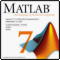Interaction between MetaTrader 4 and Matlab via CSV Files
Step-by-step instructions of how to organize data arrays exchange between MetaTrader 4 and Matlab via CSV files.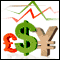Theoretical Basis of Building Cluster Indicators for FOREX
Cluster indicators are sets of indicators that divide currency pairs into separate currencies. Indicators allow to trace the relative currency fluctuation, determine the potential of formating new currency trends, receive trade signals and follow medium-term and long-term positons.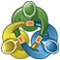Tester in the Terminal MetaTrader 4: It Should Be Known
The elaborate interface of the terminal MetaTrader 4 is a forefront, but beside this the terminal includes a deep-laid tester of strategies. And while the worth of MetaTrader 4 as a trading terminal is obvious, the quality of the tester's strategy testing can be assessed only in practice. This article shows the advantages and conveniences of testing in MetaTrader 4.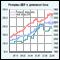Tricolor Indicators and Some Opportunities for Maximal Simplification of Writing Indicators
In this article the author dwells on some means of increasing indicators' informational value for visual trading. The author analyzes the realization of tricolor indicators, indicators, for building which data from other timeframes is used, and continues to dwell on the library of indicators, described in the article "Effective Averaging Algorithms with Minimal Lag: Use in Indicators"